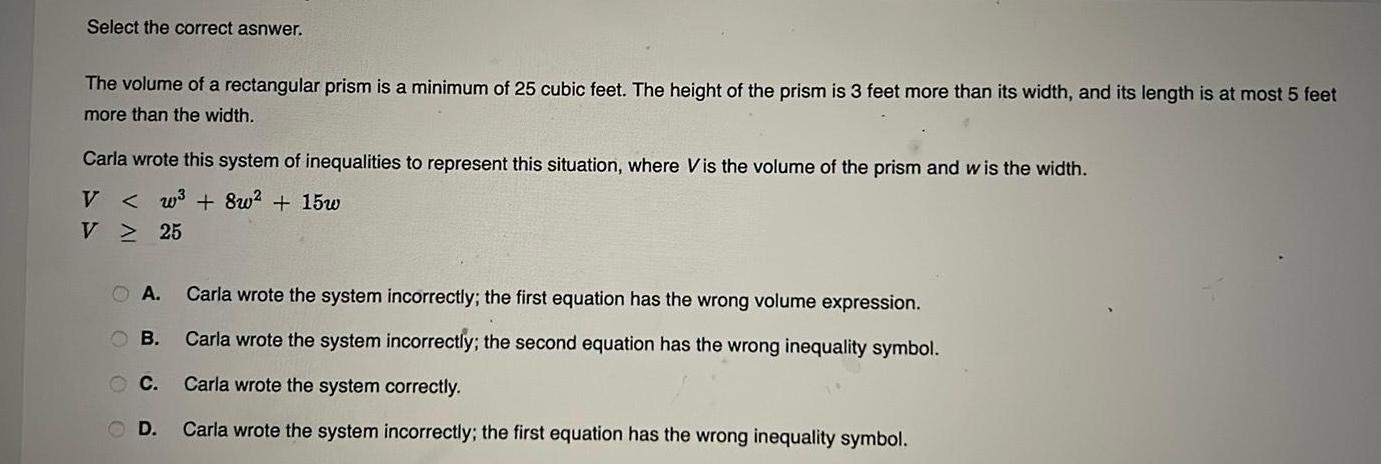Question:

# Select the correct asnwer The volume of a rectangular prism

Last updated: 11/19/2023Select the correct asnwer The volume of a rectangular prism is a minimum of 25 cubic feet The height of the prism is 3 feet more than its width and its length is at most 5 feet more than the width Carla wrote this system of inequalities to represent this situation where Vis the volume of the prism and wis the width V w 8w 15w V 25 A OB C OD Carla wrote the system incorrectly the first equation has the wrong volume expression Carla wrote the system incorrectly the second equation has the wrong inequality symbol Carla wrote the system correctly Carla wrote the system incorrectly the first equation has the wrong inequality symbol